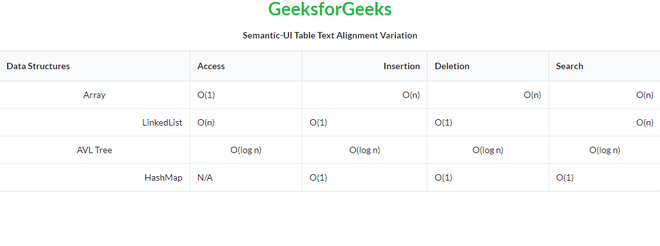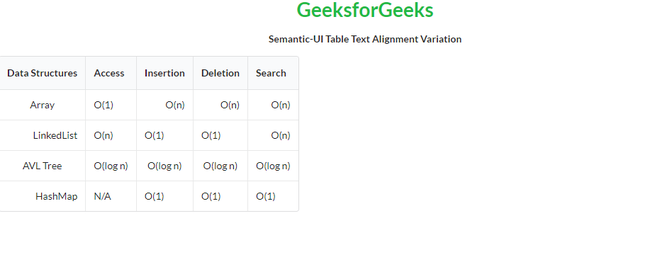GeeksforGeeks App
Open AppBrowser
Continue

# Semantic-UI Table Text Alignment Variation

Semantic UI is an open-source framework that uses CSS and jQuery to build great user interfaces. It is the same as a bootstrap for use and has great different elements to use to make your website look more amazing. It uses a class to add CSS to the elements.

Tables are an easy way to organize a lot of data. A table is an arrangement of data in rows and columns, or possibly in a more complex structure. Tables are widely used in communication, research, and data analysis. Tables are useful for various tasks such as presenting text information and numerical data. It can be used to compare two or more items in the tabular form layout. Tables are used to create databases. An HTML table and a Semantic UI table both are the same structurally.

Using Table Text Alignment variation, we can adjust the text alignment of a table header, row, or cell.

Semantic UI Table Text Alignment Variation Classes:

• right aligned: This class aligns the text on the right side.
• center aligned: This class aligns the text on the center.
• left aligned: This class aligns the text on the left side.

Syntax:

```<table class="ui table">
<tr>
<td class="right aligned">...</td>
...
</tr>
...
</table>```

Example 1: This is a basic example illustrating different Table Text Alignment variations created using Semantic UI.

## HTML

 ```<``html``>``    ``<``head``>``        ``<``title``>Semantic-UI Table Text Alignment Variation``        ``<``link` `href``=``    ``"https://cdnjs.cloudflare.com/ajax/libs/semantic-ui/2.4.1/semantic.min.css"` `              ``rel``=``"stylesheet"` `/>``      ` `    ````    ``<``body``>``        ``<``center``>``            ``<``h1` `class``=``"ui green header"``>GeeksforGeeks``            ``<``strong``>Semantic-UI Table Text Alignment Variation``        ````        ``<``table` `class``=``"ui celled table"``>``            ``<``thead``>``                ``<``tr``>``                    ``<``th``>Data Structures``                    ``<``th``>Access``                    ``<``th` `class``=``"right aligned"``>Insertion``                    ``<``th``>Deletion``                    ``<``th``>Search``                ````            ````            ``<``tbody``>``              ``<``tr``>``                ``<``td` `class``=``"center aligned"``>Array``                ``<``td``>O(1)``                ``<``td` `class``=``"right aligned"``>O(n)``                ``<``td` `class``=``"right aligned"``>O(n)``                ``<``td` `class``=``"right aligned"``>O(n)``              ````              ``<``tr``>``                ``<``td` `class``=``"right aligned"``>LinkedList``                ``<``td``>O(n)``                ``<``td``>O(1)``                ``<``td``>O(1)``                ``<``td` `class``=``"right aligned"``>O(n)``              ````              ``<``tr``>``                ``<``td` `class``=``"center aligned"``>AVL Tree``                ``<``td` `class``=``"center aligned"``>O(log n)``                ``<``td` `class``=``"center aligned"``>O(log n)``                ``<``td` `class``=``"center aligned"``>O(log n)``                ``<``td` `class``=``"center aligned"``>O(log n)``              ````              ``<``tr``>``                ``<``td` `class``=``"right aligned"``>HashMap``                ``<``td``>N/A``                ``<``td``>O(1)``                ``<``td``>O(1)``                ``<``td``>O(1)``              ````          ````        ````    `````

Output:Semantic-UI Table Text Alignment Variation

Example 2: This is a basic example illustrating different Collapsed Table Text Alignment variations created using Semantic UI.

## HTML

 ```<``html``>``    ``<``head``>``        ``<``title``>Semantic-UI Table Text Alignment Variation``        ``<``link` `href``=`` ``"https://cdnjs.cloudflare.com/ajax/libs/semantic-ui/2.4.1/semantic.min.css"` `              ``rel``=``"stylesheet"` `/>       ``    ````    ``<``body``>``        ``<``center``>``            ``<``h1` `class``=``"ui green header"``>GeeksforGeeks``            ``<``strong``>Semantic-UI Table Text Alignment Variation``        ````        ``<``table` `class``=``"ui celled collapsing table"``>``            ``<``thead``>``                ``<``tr``>``                    ``<``th``>Data Structures``                    ``<``th``>Access``                    ``<``th` `class``=``"right aligned"``>Insertion``                    ``<``th``>Deletion``                    ``<``th``>Search``                ````            ````            ``<``tbody``>``              ``<``tr``>``                ``<``td` `class``=``"center aligned"``>Array``                ``<``td``>O(1)``                ``<``td` `class``=``"right aligned"``>O(n)``                ``<``td` `class``=``"right aligned"``>O(n)``                ``<``td` `class``=``"right aligned"``>O(n)``              ````              ``<``tr``>``                ``<``td` `class``=``"right aligned"``>LinkedList``                ``<``td``>O(n)``                ``<``td``>O(1)``                ``<``td``>O(1)``                ``<``td` `class``=``"right aligned"``>O(n)``              ````              ``<``tr``>``                ``<``td` `class``=``"center aligned"``>AVL Tree``                ``<``td` `class``=``"center aligned"``>O(log n)``                ``<``td` `class``=``"center aligned"``>O(log n)``                ``<``td` `class``=``"center aligned"``>O(log n)``                ``<``td` `class``=``"center aligned"``>O(log n)``              ````              ``<``tr``>``                ``<``td` `class``=``"right aligned"``>HashMap``                ``<``td``>N/A``                ``<``td``>O(1)``                ``<``td``>O(1)``                ``<``td``>O(1)``              ````          ````        ````    `````

Output:Semantic-UI Table Text Alignment Variation

My Personal Notes arrow_drop_up
Related Tutorials